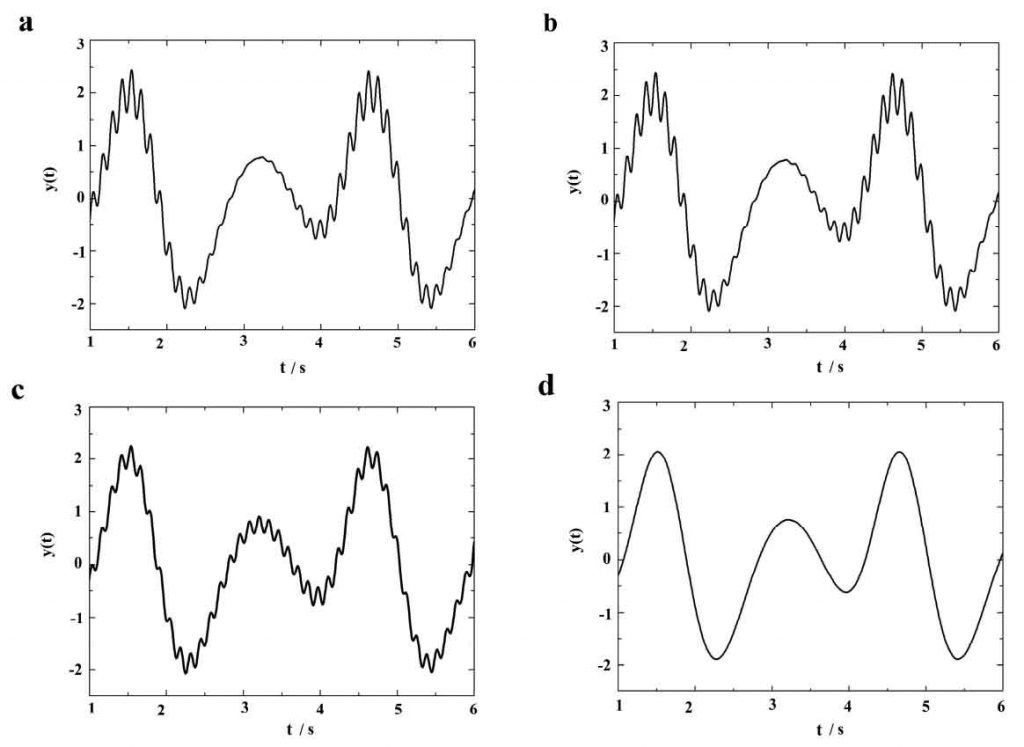# Method of determining cut off frequency in Squeeze Casting

In order to find a way to determine the truncation frequency, a function is constructed. As shown in the formula, the function contains a high-frequency noise term of 0.4sint * sin50t. The frequency of the noise term is 7.96hz, and the frequency of other terms of the function is much lower than that of the high-frequency noise term. As shown in Figure 2.8 (a), the change trend of the function in the range of 1-6s is shown. The curve clearly shows the small fluctuation caused by high-frequency noise term.(a) Unfiltered(b) The filtering result with truncation frequency of 9hz(c) The filtering result with the truncation frequency of 7.96hz(d) The filtering result with the truncation frequency of 7.7hz

According to the frequency of high-frequency noise term, three different frequencies are selected as the truncation frequency, and the low-pass filtering method based on fast Fourier transform is used to process the function. The first truncation frequency is 9hz, which is obviously higher than the noise frequency. After data processing, the result is shown in Fig. 1 (b). The result curve is basically the same as that shown in Fig. 1 (a), that is to say, it is unreasonable to use this frequency as the truncation frequency. The second truncation frequency is 7.96hz, that is, the frequency of noise term. After data processing, the result is shown in Fig. 1 (c). Although the amplitude of fluctuation is reduced, the fluctuation is not eliminated, that is to say, the noise frequency is not suitable as the truncation frequency. The third truncation frequency is 7.7hz, which is slightly lower than the noise frequency. After filtering, as shown in Figure 1 (d), the result curve is very smooth, and the fluctuation is completely removed. Therefore, the optimal truncation frequency in this example is 7.7hz.

From the above discussion, if the frequency of the noise component in the data can be determined, the truncation frequency of the optimal FFT based low-pass filtering method is slightly lower than that of the noise component. For the measurement of temperature and pressure in this experiment, the frequency of useful experimental data is far lower than that of high-frequency noise component. Therefore, as long as the frequency of the high frequency component is determined, an appropriate truncation frequency can be obtained.

As shown in Figure 2, it is the original data obtained from the measurement of temperature measurement unit and pressure sensor at position 2. Where, marked as Ta_ 1, TB_ 1 and TC_ The temperature curve of position 1 is the temperature distribution measured by thermocouples at 1 mm, 3 mm and 6 mm from the die surface at position 2. As shown in Figure 2, when the change trend of temperature slows down, the fluctuation on the temperature curve becomes more obvious, and obvious high-frequency noise signal appears. According to the technical specification of the pressure sensor, the amplitude of the pressure measurement data noise is about 1% of the sensor range, and the frequency is about 50 Hz. As shown in Figure 2, there are fluctuations in the whole pressure curve. In this experiment, the sampling frequency of the data acquisition instrument is 200Hz, so the high-frequency noise component is accurately recorded, and the frequency of the high-frequency noise component can also be obtained through the measurement data. The rectangular boxes marked a and B as shown in Figure 2 are a small part of the measured data.